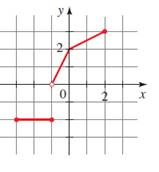# The curve is function or not &amp; the domain and range of the function.### Precalculus: Mathematics for Calcu...

6th Edition
Stewart + 5 others
Publisher: Cengage Learning
ISBN: 9780840068071### Precalculus: Mathematics for Calcu...

6th Edition
Stewart + 5 others
Publisher: Cengage Learning
ISBN: 9780840068071

#### Solutions

Chapter 2.2, Problem 56E
To determine

## To find : The curve is function or not & the domain and range of the function.

Expert Solution

The curve of the graph is the function of x and here the domain is (32) and the range is (23) .

### Explanation of Solution

Given information :Concept used : A curve in the coordinate plane is the graph of a function if and only if no vertical line intersects the curve more than once.

The domain is the set of X coordinate and the range is the set of Y coordinate of any graph.

No vertical line intersects the curve of the graph more than once, hence the curves of the graph is the function of x .

So, here the domain is (32) and the range is (23) .

### Have a homework question?

Subscribe to bartleby learn! Ask subject matter experts 30 homework questions each month. Plus, you’ll have access to millions of step-by-step textbook answers!